Test: Number Systems & Binary Codes - 2

# Test: Number Systems & Binary Codes - 2

Test Description

## 10 Questions MCQ Test Topicwise Question Bank for Electronics Engineering | Test: Number Systems & Binary Codes - 2

Test: Number Systems & Binary Codes - 2 for Electronics and Communication Engineering (ECE) 2023 is part of Topicwise Question Bank for Electronics Engineering preparation. The Test: Number Systems & Binary Codes - 2 questions and answers have been prepared according to the Electronics and Communication Engineering (ECE) exam syllabus.The Test: Number Systems & Binary Codes - 2 MCQs are made for Electronics and Communication Engineering (ECE) 2023 Exam. Find important definitions, questions, notes, meanings, examples, exercises, MCQs and online tests for Test: Number Systems & Binary Codes - 2 below.
Solutions of Test: Number Systems & Binary Codes - 2 questions in English are available as part of our Topicwise Question Bank for Electronics Engineering for Electronics and Communication Engineering (ECE) & Test: Number Systems & Binary Codes - 2 solutions in Hindi for Topicwise Question Bank for Electronics Engineering course. Download more important topics, notes, lectures and mock test series for Electronics and Communication Engineering (ECE) Exam by signing up for free. Attempt Test: Number Systems & Binary Codes - 2 | 10 questions in 30 minutes | Mock test for Electronics and Communication Engineering (ECE) preparation | Free important questions MCQ to study Topicwise Question Bank for Electronics Engineering for Electronics and Communication Engineering (ECE) Exam | Download free PDF with solutions
 1 Crore+ students have signed up on EduRev. Have you?
Test: Number Systems & Binary Codes - 2 - Question 1

### Which of the following statement is NOT correct?

Detailed Solution for Test: Number Systems & Binary Codes - 2 - Question 1

• It has a base of 16. Hence, it uses sixteen distinct counting digits 0 through 9 and A through F
• Place value (or weight) for each digit is in ascending powers of 16 for integers and descending powers of 16 for fractions.
• The chief use of this system is in connection with byte-organized machines.
• It is used for specifying addresses of different binary numbers stored in computer memory.

Complement of a Number: In digital work, two types of complements of a binary number are used for complemental sub-traction:
1’s complement:

• The 1’s complement of a binary number is obtained by changing each 0 into a 1 and each 1 into a 0.
• It is also called radix-minus-one complement.
• For example, 1’s complement of 1002 is 0112, and 0112 is 00012.

2’s complement:

• The 2’s complement of a binary number is obtained by adding 1 to its 1’s complement.
• 2’s complement = 1’s complement + 1
• It is also known as a true complement.

Octal Number System:

• It has a base of 8 which means that it has eight distinct counting digits: 0, 1, 2, 3, 4, 5, 6, and 7
• These digits 0 through 7, have precisely the same physical meaning as in the decimal system.
• For counting beyond 7, 2-digit combinations are formed taking the second digit followed by the first, then the second followed by the second, and so on.
• Hence, after 7, the next octal number is 10 (second digit followed by first), then 11 (second digit followed by second), and so on.

Excess-3 Code:

• It is an unweighted code and is a modified form of BCD.
• It is widely used to represent numerical data in digital equipment.
• It is abbreviated as XS-3. As its name implies, each coded number in XS-3 is three larger than in the BCD code.
Test: Number Systems & Binary Codes - 2 - Question 2

### Conversion of (98.75)10 into binary, octal and hexadecimal number system, respectively, is:

Detailed Solution for Test: Number Systems & Binary Codes - 2 - Question 2

Concept:
Conversion of decimal to binary:

Step 1: Divide the number by 2 keeping notice of the quotient and the remainder. Continue dividing the quotient by 2 until you get a quotient of zero.
Step 2: Write out the remainders in the reverse order to get the equivalent binary number.

For converting decimal fractions to binary numbers, follow these steps:
For converting decimal fractions to a binary numbers, follow these steps:
Then write out the integer parts from the results of each multiplication to get the equivalent binary number.

Conversion of binary to octal:
Make pair of three binary number which forms an octal number.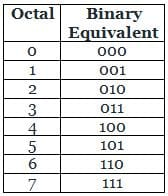Make pair of four binary number which forms a hexadecimal number.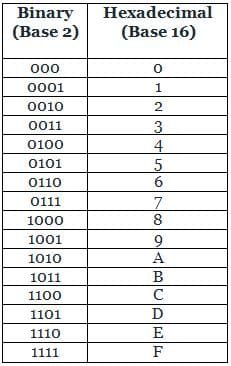Calculation:
Given, that the decimal number = (98.75)10
98 / 2 = 49 with remainder 0
49 / 2 = 24 with remainder 1
24 / 2 = 12 with remainder 0
12 / 2 = 6 with remainder 0
6 / 2 = 3 with remainder 0
3 / 2 = 1 with remainder 1
1 / 2 = 0 with remainder 1

Write in reverse order:
98 = 1100010
0.75 × 2 = 1 + 0.5
0.5 × 2 = 1 + 0
.75 = .11
(98.75)10 = (1100010.11)2
(98.75)10 = (001  100  010.  110)2 = (142.6)8 ...........(from table 1)
(98.75)10 = (0110  0010.  1100)2 = (62.C)16...........(from table 2)

Test: Number Systems & Binary Codes - 2 - Question 3

### Convert the 127 decimal number into binary.

Detailed Solution for Test: Number Systems & Binary Codes - 2 - Question 3

Concept
Divide 127 by 2. Use the integer quotient obtained in this step as the dividend for the next step. Repeat the process until the quotient becomes 0.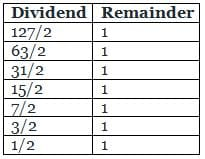Write the remainder from bottom to top i.e. in the reverse chronological order.
This will give the binary equivalent of 127.
Therefore, the binary equivalent of decimal number 127 is 1111111.

Test: Number Systems & Binary Codes - 2 - Question 4

The 2’s complement of 1010101 is ______.

Detailed Solution for Test: Number Systems & Binary Codes - 2 - Question 4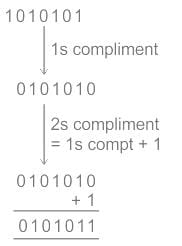Tips and Tricks:
Steps to writing 2’s complement to any binary number:

• Start from right to left and search for the first ‘1’
• Write down the bits until that first ‘1’ as it is.
• Write down the remaining left bits with their respective complement.

Ex. Let the given number is: 100100
The 2’s complement is as shown.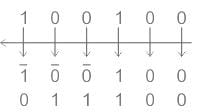Given number is 1010101
2’s complement = 0101011

Test: Number Systems & Binary Codes - 2 - Question 5

The binary code of (21.125)10 is

Detailed Solution for Test: Number Systems & Binary Codes - 2 - Question 5

Step 1: Divide (21)10 successively by 2 until the quotient is 0.
21/2 = 10, remainder is 1
10/2 = 5, remainder is 0
5/2 = 2, remainder is 1
2/2 = 1, remainder is 0
1/2 = 0, remainder is 1

Step 2: Read from bottom (MS2) to top (LS2) as 10101
This is the binary equivalent of decimal number 21

Step 3: Binary equivalent of 0.125 is, multiplying by 2 until we get 1 and writing down the integer after each multiplication,
⇒ 0.125 × 2 = 0.25
⇒ 0.25 × 2 = 0.5
⇒ 0.5 × 2 = 1
⇒ Binary equivalent of 0.125 = 001
∴ The binary code of (21.125)10 is,
(21.125)10 = 10101.001

Test: Number Systems & Binary Codes - 2 - Question 6

The decimal equivalent of the binary number (1101)2 is

Detailed Solution for Test: Number Systems & Binary Codes - 2 - Question 6

Step by step solution:

• Step 1: Write down the binary number: 1101
• Step 2: Multiply each digit of the binary number by the corresponding power of two: 1x23 + 1x22 + 0x21 + 1x20
• Step 3: Solve the powers: 1x8 + 1x4 + 0x2 + 1x1 = 8 + 4 + 0 + 1
• Step 4: Add up the numbers written above: 8 + 4 + 0 + 1 = 13
• So, (1101)2 = (13)10
Test: Number Systems & Binary Codes - 2 - Question 7

The hexadecimal representation of 6578 is

Detailed Solution for Test: Number Systems & Binary Codes - 2 - Question 7

Concept:
Hexadecimal number: In this, value of the base is 16. Each digit is represented by 4-bit binary no.
Octal number: For octal number, value of base is 8. Each digit of an octal number is represented by 3-bit binary no.
Octal number = 657
Binary representation for this number (each digit of a octal number is converted into 3 binary bits)
So, 657 in binary is equivalent to 110 101 111
Now group this binary number into 4 bits starting from right to left.
i.e. 0001 1010 1111
Hexadecimal representation for this number is : 1AF

Test: Number Systems & Binary Codes - 2 - Question 8

Find the decimal equivalent of the 6-bit binary number (101.101)2

Detailed Solution for Test: Number Systems & Binary Codes - 2 - Question 8

The decimal equivalent of the binary number 101.101 is,
= 1 × 22 + 0 × 21 + 1 × 20 + 1 × 2-1 + 0 × 2-2 + 1 × 2-3
= 4 + 0 + 1 + 0.5 + 0 + 0.125
= 5.625

Test: Number Systems & Binary Codes - 2 - Question 9

The decimal number (57.375)10 when converted to binary number takes the form:

Detailed Solution for Test: Number Systems & Binary Codes - 2 - Question 9

Concept:
Decimal to binary:

• Take decimal number as dividend.
• Divide the number by 2.
• Get the integer quotient for the next iteration.
• Get the remainder (it will be either 0 or 1 because of divisor 2).
• Repeat the steps until the quotient is equal to 0
• Write the remainders in reverse order (which will be equivalent binary number of given decimal number).

Decimal to binary: (fractional part)

• Take decimal number as multiplicand.
• Multiple this number by 2 (2 is base of binary so multiplier here).
• Store the value of integer part of result in an array (it will be either 0 or 1 because of multiplier 2).
• Repeat the above two steps until the number became zero.
• Write these resultant integer part

Calculation:
Binary of  57: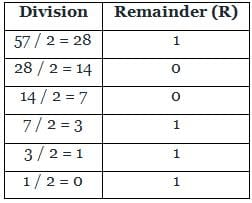Now, write remainder from bottom to up (in reverse order), this will be 111001 which is equivalent binary number of decimal integer 57.
Convert decimal fractional number 0.375 into binary number.
Here, decimal fraction: 0.375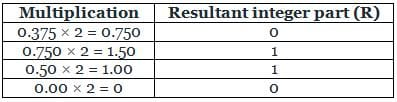Now, write these resultant integer part, this will be 0.0110 which is equivalent binary fractional number of decimal fractional 0.375.
∴ 57.375 can be written as 111001.011 in binary
Hence, option (1) is correct.

Test: Number Systems & Binary Codes - 2 - Question 10

The range of numbers represented by an 8-bit two’s complement representation is

Detailed Solution for Test: Number Systems & Binary Codes - 2 - Question 10

The range of n bit word in 2’s complement representation is,
(−2n−1) to (2n−1 − 1)
(n-1) is used here because out of n bits 1 bit is used as a sign bit
There is one extra negative number because "0" has only a single representation in 2's complement form
For 8 bit word, the range will be -128 to 127.

## Topicwise Question Bank for Electronics Engineering

193 tests
Information about Test: Number Systems & Binary Codes - 2 Page
In this test you can find the Exam questions for Test: Number Systems & Binary Codes - 2 solved & explained in the simplest way possible. Besides giving Questions and answers for Test: Number Systems & Binary Codes - 2, EduRev gives you an ample number of Online tests for practice

## Topicwise Question Bank for Electronics Engineering

193 tests(Scan QR code)